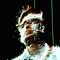# Recurrent Neural Networks in Ruby

We look at how neural networks work, what is different about a recurrent networks and a library which allows us to use recurrent networks in Ruby (tlearn-rb).

## What the heck is a Recurrent Network?

First lets look briefly at how a neural network works:

## Neural Networks

Neural networks use the model of neurones in the human brain. Put very simply the artifical neuron given some inputs (the dendrites) sums them to produce an output (the neuron’s axon) which is usually passed through some non-linear function. The sum of the nodes is usually weighted.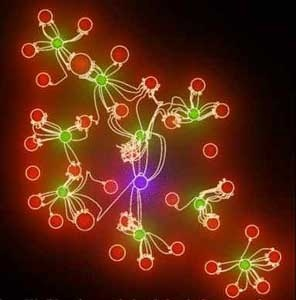By taking a set of training data we can teach a neural network such that it can be applied to new data outside of the training set. For example we could have as inputs the states of a chess board and the output as a rank for how good the position is for white. We could after training, input an unseen board state and as output get a rank for how effective the position is for white.

As a neural network is trained it builds up the set of weights for the connections between nodes. Through many training iterations comparing expected outputs and the inputs these weights are built up.

#### Feedforward Neural Network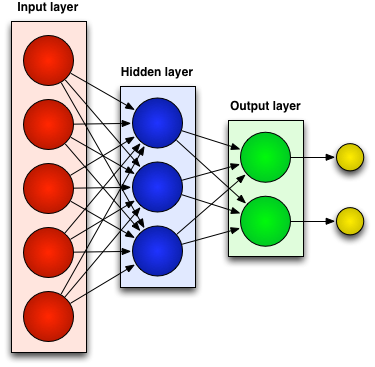In some problems the order in which the inputs arrive at the network is important. A normal network fails at this as there is no explicit sense of the relationships between sets of inputs.

Lets consider an example. A network that is trained to detect how satisfying a word sounds to children.

We feed our network all the syllables of a word and get an output:

``````["mon", "key"]
["o", "key", "do", "key"]
``````

When we feed the syllable “key” into the neural network it will always return the same output irrelevant of what came before it. This misses a relationship between the syllables of the word.

A recurrent network aims to solve this problem by using both the input layer and the output layer to devise weights of the hidden layer.

#### Recurrent Network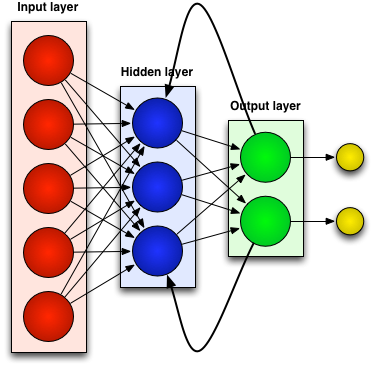Going back to our example:

``````["mon", "key"]
["o", "key", "do", "key"]
``````

When we feed “key” into the neural network the weight returned will be effected by what the previous input was, [“mon”] or [“o”, “key”, “do”].

So our recurrent neural network would detect that “o-key-do-key” has a rhythm between the syllables that is appealing to children.

A recurrent network allows us to decided when to wipe the previous output and start again. So in our example we would reset the output layer after we have fed in all the syllables of the word. We are interested in the relationships between syllables of a word, not syllables of different words.

So all this is a complicated way of saying Recurrent networks have state. Yes.

## Recurrent Networks in Ruby

There was no Ruby library that support Recurrent Networks. There was an attempt to add Recurrent networks to FANN (which has a ruby-fann gem with bindings) but it was never merged in.

So I adapted the TLearn C library which supports Recurrent Neural Networks and wrapped it in Ruby Love.

It’s having some trouble coming to terms with its new found rubyness, so there is a big alpha warning hanging on the door.

### Installing TLearn

``````gem install tlearn
``````

### Wait! What does that output mean?

In our example we had 2 outputs. The result we get from running the fitness test are the final weights:

``````[0.2, 0.9]
``````

In this example we can think of the first output as rank 1, and the second output as rank 2. We look at which has the highest weighting in the fitness test, In this case it shows us that the input “000” has rank 2. So really we can map the output to many different classifications.

### How is state reset?

Tlearn resets the state for each list of elements

### Wait! What the heck does all that config mean?

Part of the work of using Neural networks is finding the right configuration settings. TLearn supports a lot of different options. Lets look at what all that configuration options means. (Checkout the TLearn Github Readme for full details of the config options):

``````:number_of_nodes => 10
``````

The total number of nodes in this network (not including input nodes)

``````:'output_nodes'    => 5..6
``````

Which nodes are used for output.

``````:linear          => 7..10
``````

Nodes 7 to 10 are linear. This defines the activation function of the nodes. The activation function is how all the weights and input are combined for a node to create an output. Linear nodes output the inner-product of the input and weight vectors.

``````:weight_limit    => 1.00
``````

Limit of 1.0 must not be exceeded in the random initialization of weights.

#### Connections

Connections specify how all the nodes of the neural network connect. This is the architecture of the neural network. Lets look at the connection settings:

``````{1..6   => 0}
``````

Node 0 feeds into node 1 to 6. Node 0 is the bias node that is always 1.`````` {1..4   => :i1..:i3}
``````

The input nodes 1-3 feed into each node from 1 to 4.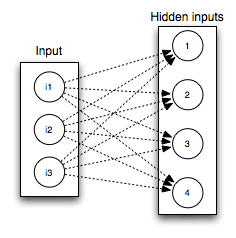``````{1..4  => 7..10},
``````

Nodes nodes 7-10 feed into each node from 1 to 4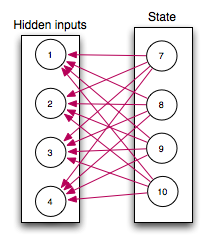``````{5..6   => 1..4},
``````

Nodes nodes 1..4 feed into each node from 5 to 6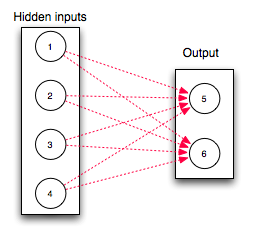`````` {7..10  => [1..4, {:min => 1.0, :max => 1.0}, :fixed, :'one_to_one'}]
``````

This connection contains a couple of special options. Rather than node 1-4 being fed into node 7, node 1 only connects with node 7, node 2 only with node 8, node 3 only with node 9, node 4 only with node 10. The :‘one_to_one’ option causes this. The weights of the connections between these nodes is fixed at 1.0 and never changes throughout training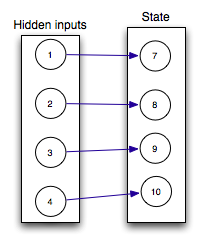So put all these together our full neural network is: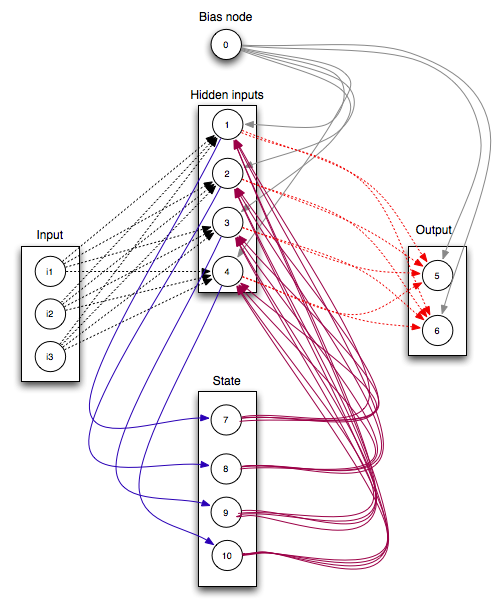### Urmm… So how do I know what connection settings to use?

When it comes to deciding how many hidden nodes to have in your network there is a general rule:

The optimal number of hidden nodes is usually between the size of the input and size of the output layers

When deciding what connections to specify in your neural network you can start with everything connected to everything and slowly experiment with pruning connections/nodes which will increase the performance of your network without radically affecting the output efficiency.

Its important to have the bias node connect to all the nodes in the hidden layer and output. This is required so a zero input to the neural network can generate outputs other than 0.

With recurrent networks it is important to build connections and nodes in your network to maintain state. It is quite possible with TLearn to build a plain old neural network with no state. It can be helpful like the example given above to draw out your state, hidden layer and output layer nodes and use this to decided how the network connects.

How do you decide what activation functions to use? Linear, bipolar, etc. Checkout this great paper on the effectiveness of different functions: http://www.cscjournals.org/csc/manuscript/Journals/IJAE/volume1/Issue4/IJAE-26.pdf

One neat (crazy) experimental (crazy) path to explore is neural network toplogies generated from using a genetic algorithm to assess the effectiveness of the network: http://www.cs.ucf.edu/~kstanley/neat.html.

### TLearn’s Source

If you want to peer into the heart of TLearn the source code is on Github:

``````git clone git://github.com/josephwilk/tlearn-rb.git
``````